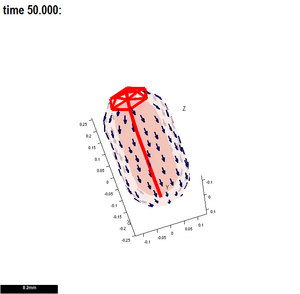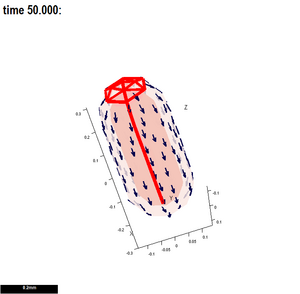# Difference between revisions of "GFtbox Tutorial pages"

The models shown in these tutorials illustrate features of the GFtbox software. They are not designed to understand the Growing Polarised Tissue Framework which is better done with Examples from our papers.

# Three ways to use GFtbox

1) Do everything from the Graphical User Interface (GUI). See here for the best way to start working with GFtbox

2) Do only some things from the GUI. See here for the best way to develop ideas on the growth of shape. Use the GUI to generate the mesh (canvas) and create growth factors (morphogens - in other words declare the variables) but capturing your ideas on how the regulatory processes work in, what we call, the interaction function.

For an introduction to the output and results, see here.

3) Without the GUI. Running models without the GUI, see here. For example, run many examples (instances) of a pre-existing project on a cluster. This is the best way to explore the parameter space of a model for comparison with biological observations. We use this extensively once we have roughed out the basic ideas of a model interactively.

# 1 Modelling using the Graphical User Interface

## Isotropic growth

How to use the tutorial. Open the GFtbox and attempt to repeat the results shown. There are notes to explain how to keep a record of your results, e.g. Snapshots, Movie.

### 1 A

 For tutorial on uniform growth click here.Consider a disc shaped canvas (tissue) in which the specified growth is uniform, isotropic and on both sides.Into what shape will the disc grow?This model is as simple as it gets. Notice that, during growth, the mesh is automatically subdivided. Notice also that the final surface is not quite flat. This is because, to allow it to deform in 3D, it is not flat initially. There are options to initialise a flat mesh and others to force it to remain flat - see options on the GUI (hover over controls to get prompts). In the absence of a polariser (there is no polariser in this example) growth will be isotropic, in other words growth in the plane of the canvas will be the average of what is specified for Kapar and Kaper (A side) and Kbpar and Kbper (B side). GPT_tut_uniform_20110527-0003.flv|GPT_tut_uniform_20110527-0003_Last.png

### 1 B

 For tutorial on non-uniform growth click hereConsider a disc shaped canvas (tissue) in which the non-uniform specified growth increases in proportion to the distance from the centre.Into what shape will the disc grow?Already we are into the realms of modelling biological systems. Compare this result with the discussion of Lily petals and Gaussian curvature (Lianga and Mahadevana,Sharon, Marder and Swinney,Nath, Crawford, Carpenter and Coen ). GPT_tut_uniform_20110527-0006.flv|GPT_tut_uniform_20110527-0006_First.png

### 1 C

 For tutorial on uniform growth non-uniform polariser click here In the presence of polariser, GFtbox growth will be anisotropic, in other words growth in the plane of the canvas can be different parallel and perpendicular to the axis of the polariser: Kapar and Kaper (A side) and Kbpar and Kbper (B side). We now add polariser. Start with example A - uniform growth - and add a radial polarising gradient.Arrows show the direction of the gradient. They are turned on using the Panel:Plot option:Pol. grad. tick box. The gradient defines local directions and local growth can be specifed either parallel to (Kpar) or perpendicular to (Kper) that direction. Given a uniform pattern of specified growth parallel to the polariser and zero specified growth perpendicular to the polariser. What will be the final shape? GPT_in_the_beginning_2_20110510-0003.flv|GPT_in_the_beginning_2_20110510-0003_First.pngNote: the gradient of the polariser, green to cyan, is shown using the arrows. Specified growth rate parallel to the arrows, red, is uniform.

# 2 Modelling using a combination of GUI and interaction function

Programmatic modelling.

How to use these tutorials. Use a combination of the GUI to set up the Mesh structure and editing the associated interaction function to repeat the results shown. Full listings of the interaction functions are given from which you can copy the key, editable, elements.

The full specification of a GFtbox model is stored in a combination of the mesh data structure (Mesh) and the interaction function. The Mesh stores all the physical properties of the system: spatial structure, mechanical properties, etc. It is usually set up using the GUI. The Mesh is stored on disc as a Matlab data file (.mat) and in memory as a data structure (m). The interaction function (a Matlab program file .m) contains all the details of the growth regulation system: morphogen concentrations, signal interactions etc.

When a new project is first edited the interaction function is generated automatically. Thereafter, it is automatically kept in synchrony with the GUI. It is divided into several sections. Some are generated automatically and should not be edited. Others are set aside for the user to specify the model. To ensure that the automatic and manual edits are synchronised always invoke the Editor from the GUI (Panel: Interaction Function: Edit).

### 2 A

Basic interaction function The patterns of morphogens A and B are set up by

```       id_a_p(m.nodes(:,1)<-0.03)=1;
id_b_p(m.nodes(:,2)<-0.01)=1;
```

where id_a_p is the A morphogen. m.node(:,1) refers to the x coordinates of all nodes (vertices) in the mesh
The expression (m.nodes(:,1)<-0.03) means find all vertices with x coordinates that are less than -0.03.

Similarly, (m.nodes(:,2)<-0.01) means find all vertices with y coordinates that are less than -0.01.

And the pattern of polariser (P) is set up by

```       P((m.nodes(:,1)<-0.05)&(m.nodes(:,2)>0.03))=1;
```

Where (m.nodes(:,1)<-0.05)&(m.nodes(:,2)>0.03) means find all vertices with x,y coordinates that are less than -0.05 and greater than 0.03 respectively

Thus the full code describing the model is:

```   if (Steps(m)==0) && m.globalDynamicProps.doinit  % Initialisation code.
id_a_p(m.nodes(:,1)<-0.03)=1; % setup region for A where identity factor A is represented by id_a_p
id_b_p(m.nodes(:,2)<-0.01)=1; % setup region for B
else
% @@KRN Growth Regulatory Network
kapar_p(:) = id_a_l .* inh(1,id_b_l); % growth rate
kaper_p(:) = kapar_p; % isotropic growth
kbpar_p(:) = kapar_p; % same on both sides of the sheet
kbper_p(:) = kapar_p; % same
knor_p(:)  = 0;       % thickness not growing
end
```
 For tutorial on a basic interaction function click here The tutorial explains how the code shown above appears in the interaction function. To create the interaction function, first create and save a project (see above) then click Panel:Interaction function:Edit. The interaction function will contain all the default variables and any patterns of variables (Kapar, etc.) that have already been set through the GUI. Types of morphogens and factors are given here. GPT_why_matlab-2011-05-05-0005.flv|GPT_why_matlab-2011-05-05-0005_First.png‎ Play and the mesh will grow and subdivide. Normally we do not want to see the mesh. Red is growth rate

### 2 B

Interaction function in detail

(A newly created interaction function is shown here - this one has two, user specified morphogens: id_a and id_b.)

There are two submodels. An identical growth pattern and polariser pattern is specified in both, but only the specified growth in the second is anisotropic and so uses the axiality specified by the polariser gradient. The gradient is zero in the region where the polariser is held at a constant value of one - and therefore growth in the region without arrows is isotropic.

 Tutorial on the interaction function details click here GPT_tut_interaction_example_20110601-0002.flv|GPT_tut_interaction_example_20110601-0002.png‎ Play submodel 1 Submodel 2. Growth in the region that has no arrows is isotropic and, as result, the outgrowth is blunt. GPT_tut_interaction_example_20110601-0007.flv|GPT_tut_interaction_example_20110601-0006_Last.png‎ Play submodel 2

# Output and Results

### 2 C Illustrating two independent ways to form shapes and the use of submodels.

Conclusion: interesting shapes can be generated either by patterns of differential growth or patterns of local growth axes.

Tutorial on two romatic hearts click here

 Submodel 1. Uniform specified polariser (no polariser gradient). Creating a shape using a specified pattern of isotropic growth. Result: simple patterns of differential growth can readily produce blobby shapes. GPT_TwoWayHeart_20110531-0001.flv|GPT_TwoWayHeart_20110531-0001_Last.png‎ Play submodel 1. Pattern of isotropic specified growth (no polariser) Submodel 2.Uniform specified growth. Creating a shape using a specified pattern of diffusable polariser. Result: simple patterns organising a diffusable polariser can readily produce sharp shapes. GPT_TwoWayHeart_20110531-0002.flv|GPT_TwoWayHeart_20110531-0002_Last.png‎ Play submodel 2. Pattern of specified polariser levels (green-cyan). Polariser can diffuse and the gradient is arrowed. Uniform specified growth (red).

### 2 D

Retaining strain and cutting the mesh

There are two submodels. They are the same except that in the first strain is not retained from step to step whereas in the second strain is fully retained. The shape changes during growth look similar, the difference is only revealed when the mesh is cut. The behaviour of plant tissue can be similar.

Tutorial on retaining residual strain and cuttingStrain not retained after each step. In plant tissue terms this would appear to be equivalent to there being a homeostatic mechanism that operates such as to re-strengthen the cell walls after they have been weakened to the point that they yield under turgor pressure during growth. GPT_RetainStrainAndCut_20110603-0006.flv|GPT_RetainStrainAndCut_20110603-0006.png‎ Play submodel 1Retaining the strain from step to step allows the growth on the inside to force the mesh as a whole to grow more than when strain is not retained. GPT_RetainStrainAndCut_20110603-0004.flv|GPT_RetainStrainAndCut_20110603-0004.png‎ Whilst the frame rate relates directly to growth rate, the relaxation rate after cutting does not. After cutting the computation has to be done in smaller steps and the rate at which the sides bend back shown here is too slow.

# 3 Running models without the GUI

Having developed the concepts underpinning a pattern of growth in an interaction function it is often desirable to explore a range of model parameters. Given that each run of the model can take between 5 minutes and hour it is appropriate to run the models in parallel on a computing cluster. (Each node of the cluster to be used by GFtbox needs to be licensed to run Matlab - however for the purpose of running on a cluster without the GUI we are exploring the possibility of making GFtbox compatible with Octave.)

How to use these tutorials. First ensure that your model is working as you would expect using the GUI. Then run the model from the Matlab command line. Finally, submit the project to the linux computing cluster with one or more ranges of parameters. For further details Running example models and using a cluster